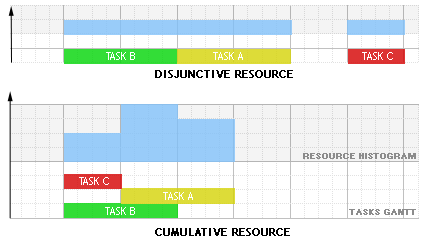# KCumulativeResourceConstraint¶

class KCumulativeResourceConstraint : public KConstraint

This constraint states that some tasks requiring a resource do not exceed the resource capacity. The primary use of this constraint is to express resource constraints.

Resources (machines, raw material etc) can be of two different types:

• Disjunctive when the resource can process only one task at a time (represented by the class KUnaryResource).

• Cumulative when the resource can process several tasks at the same time (represented by the class KDiscreteResource).

Traditional examples of disjunctive resources are Jobshop problems, cumulative resources are heavily used for the Resource-Constrained Project Scheduling Problem (RCPSP). Note that a disjunctive resource is semantically equivalent to a cumulative resource with maximal capacity one and unit resource usage for each task using this resource but this equivalence does not hold in terms of constraint propagation. The size of a task is its duration by its usage: use_i * dur_i = size_i.

The following schema shows an example with three tasks A,B and C executing on a disjunctive resource and on a cumulative resource with resource usage 3 for task A, 1 for task B and 1 for task C :Since

2016.1

Public Functions

KCumulativeResourceConstraint(KProblem &p, KIntVarArray &starts, KResource_I *r, int propagation = KDiscreteResource::TasksIntervals)

Constructor for internal use only

KCumulativeResourceConstraint(KProblem &p, KIntVarArray &starts, KIntVarArray &ends, KIntVarArray &durs, KIntVarArray &uses, KIntVarArray &sizes, int C, int propagation = KDiscreteResource::TasksIntervals)

Constructor

See

Parameters
• p – the problem

• starts – the array of variables representing the starts of the tasks

• ends – the array of variables representing the ends of the tasks

• durs – the array of variables representing the durations of the tasks

• uses – the array of variables representing the consumptions of the tasks

• sizes – the array of variables representing the sizes of the tasks

• C – initial resource stock capacity

• propagation – propagation scheme

KCumulativeResourceConstraint(KProblem &p, KIntVarArray &starts, KIntVarArray &ends, KIntVarArray &durs, KIntArray &prodsidx, KIntVarArray &prods, KIntVarArray &sizesp, KIntArray &consosidx, KIntVarArray &consos, KIntVarArray &sizesc, int C, int propagation = KDiscreteResource::TasksIntervals)

Constructor

See

Parameters
• p – the problem

• starts – the array of variables representing the starts of the tasks

• ends – the array of variables representing the ends of the tasks

• durs – the array of variables representing the durations of the tasks

• uses – the array of variables representing the consumptions of the tasks

• sizes – the array of variables representing the sizes of the tasks

• C – initial resource stock capacity

• propagation – propagation scheme

KCumulativeResourceConstraint(const KCumulativeResourceConstraint &toCopy)

Copy constructor